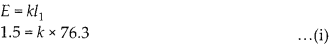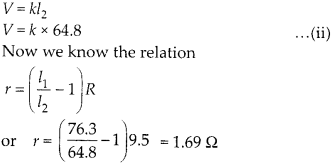Enlightened

# Question 24: NCERT Solutions for 12th Class Physics: Chapter 3-Current Electricity

• 0

Question 24: NCERT Solutions for 12th Class Physics: Chapter 3-Current Electricity

Figure shows a 2.0 V potentiometer used for the determination of internal resistance of a 1.5 V cell. The balance point of the cell in open circuit is 76.3 cm. When a resistor of 9.5 Ω is used in the external circuit of the cell, the balance point shifts to 64.8 cm length of the potentiometer wire. Determine the internal resistance of the cell.Share

1. Solution:
In the open circuit, the balance point is obtained for the emf of 1.5 V.When the external circuit is connected, a current is drawn from the cell of 1.5 V in external resistance of 9.5 E1. Now the balance point is obtained for terminal potentialCheck the complete chapter with solutions.

NCERT Solutions for 12th Class Physics: Chapter 3-Current Electricity

• 0